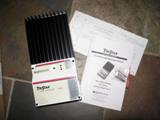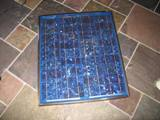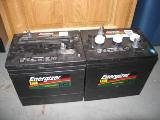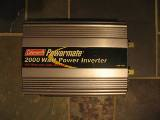• Home
• Batteries
• Charge Controllers
• DC Disconnect
• FAQ
• Forum
• Photos
• Power Inverters
• Test Power Inverter
• Inverter Efficiency
• Modified Wave vs. Pure Sine Wave
• Inverter Surge vs. Continuous Watts
• Safety
• Solar Panels
• Tower
• Videos
• Wiring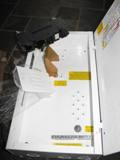-Storage

#### Other Alt Power:

• Bicycle Generator

### Encyclopedia of Alternative Energy:

amp (ampere): - An amp (ampere) (A) is a basic unit of electrical current. You will hear this refered when you are asking how many amps does a light bulb draw, and ect..

a simple equation dealing with amp/ampere is: a 60 watt light bulb running on your common household line running a 110 volts pulls around .54 amperes.

watts / voltage = amps(amperes). (60/110=.5454...)

Amp Hour - An Amp-hour is a rating that is given to a battery to tell you how much amperage is available when you discharge it evenly over a 20-hour period(commonly). This amp-hour rating is also a cumulative rating, to figure out how many amps the battery will actually output for 20 hours you divide this amp hour rating by 20.

for example: you have a 220 amp hour battery, to get the standard amp hour rating you would take 220/20 = 11 amps for 20 hours.

Battery: - A battery is basically a storage container for electricity. A rechargeable/deep cycle type battery can be charged and discharged many times. Batteries are not 100% animals, they take more energy to charge than they will actually hold. When charging a battery you have a chemical reaction between the materials in the battery, these materials give off heat in turn using some of the energy making the chemical reaction. The slower the battery is charged/discharged the more efficient a battery is. There are many types of batteries on the market today, some common batteries are:

Starting Batteries - commonly found in cars/trucks, these batteries send a surge stored energy to the starter of a vehicle to turn the engine over to initially start the car/truck.(not good for alternative energy projects)

Deep Cycle Batteries - commonly used for alternative energy projects, this category including Lead-acid, and Gel type rechargable batteries. These types of batteries will commonly have an amp hour rating on them.(great for alternative energy projects!)

Marine Batteries - sometimes can be a deep cycle battery, but these types of batteries are more commonly a hybrid battery. They mostly contain spongelike lead plates, and cannot be discharged more than 50% capacity. Were as a deep cycle can be discharged 80%.(not good for alternative energy projects)

Kilowatt: - A Kilowatt (kW) is equal to 1000 watts. Kilowatt is generally used to state the output of larger wind generators and motors alike. Not to be confused with Kilowatt Hour.

Kilowatt Hour: - A Kilowatt Hour(kWh) is commonly seen on something like your powerbill. Utility companies charge a rate per Kilowatt-Hour used. What a Kilowatt Hour basically means is the amount of energy used up if work is done for a constant hour at a rate of 1000 watts. 1000 watts constantly used in an hour period = 1 Kilowatt-hour (kWh).

Lets look at the equation to figure out Kilowatt Hours:

amps X voltage = watts

watts / 1000 = kilowatts

kilowatts x amount of time something is run = Kilowatt Hours

Solar Panel: - A Solar Panel is a thin panel consisting of an array of solars cells connected together to create a specified voltage that is turned into electricity. These panels are put on rooftops, satellites, and setup on solar farms to provide electricity for many applications. Typically these panels are used in alternative energy projects to collect energy to recharge batteries, run attic fans, water fountains, and ect,..

Watt: - A Watt(W) is used in stating the energy used of things like light bulbs and lots of common household and mechanical energy consuming products. For example: a regular incandescent light bulb uses electricity at a rate of anywhere from 25-100 watts typically. Today we have compact florescents that consume considerably less watts.

Lets look at the equation to figure out watts:

amps X voltage = watts

example: a light bulb that pulls .5 amps X 120 volts(common household electric) = 60 watts. (.5 X 120 = 60)

Watt-hour: - A Watt-hour is expressed by giving the watts a load uses for 1 hour. for example: a 60watt incandescant light bulb uses 60watts over an hours time period.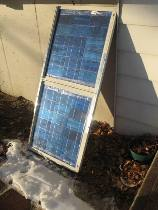#### Latest News:

Working on the new DIY Solar panel build pages! Hope to have them up soon, lots of images and tips and tricks!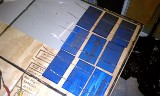#### Encyclopedia of Alternative Energy:

Amp

Amp Hour

Battery

Kilowatt

Kilowatt Hour

Solar Panel

watt